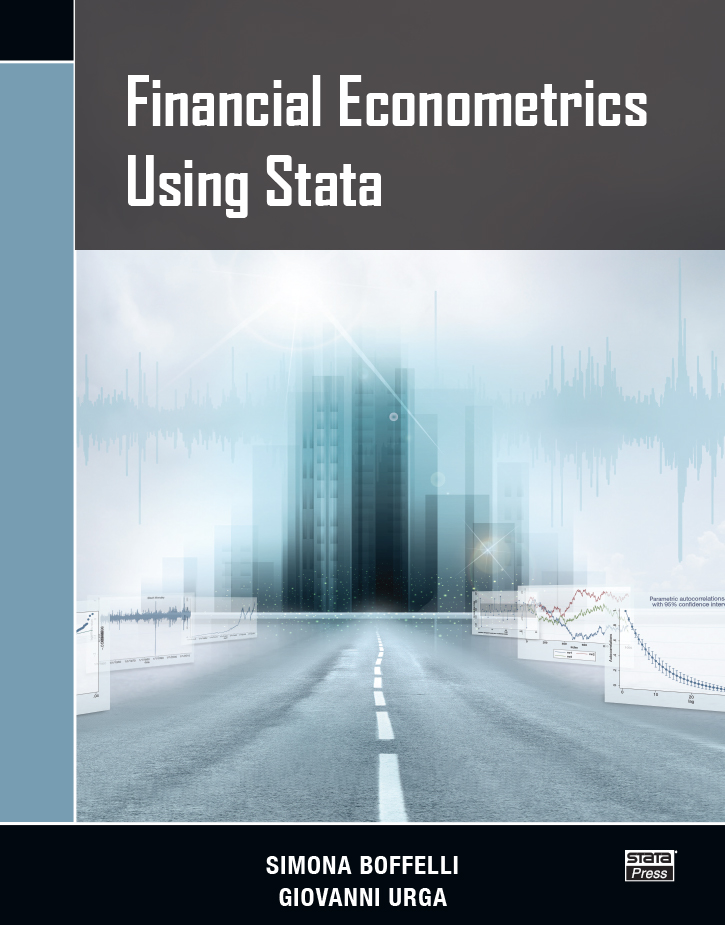# Financial Econometrics Using Stata

Financial Econometrics Using Stata by Simona Boffelli and Giovanni Urga provides an excellent introduction to time-series analysis and how to do it in Stata for financial economists. Aimed at researchers, graduate students, and industry practitioners, this book introduces readers to widely used methods, shows them how to perform these methods in Stata, and illustrates how to interpret the results.

After providing an intuitive introduction to time-series analysis and the ubiquitous autoregressive moving average (ARMA) model, the authors carefully cover univariate and multivariate models for volatilities. Chapters on risk management and analyzing contagion show how to define, estimate, interpret, and perform inference on essential measures of risk and contagion.

The authors illustrate every topic with easily replicable Stata examples and explain how to interpret the results from these examples.

The authors have a unique blend of academic and industry training and experience. This training produced a practical and thorough approach to each of the addressed topics.

List of figures
Preface
Notation and typography

1.INTRODUCTION TO FINANCIAL TIME SERIES

The object of interest

Approaching the dataset
Normality
Stationarity

Stationarity tests

Autocorrelation

ACF
PACF

Heteroskedasticity
Linear time series
Model selection
How to import data

2. ARMA MODELS

Autoregressive (AR) processes

AR(1)
AR(p)

Moving-average (MA) processes

MA(1)
MA(q)
Invertibility

Autoregressive moving-average (ARMA) processes

ARMA(1,1)

ARMA(p,q)
ARIMA
ARMAX

Application of ARMA models

Model estimation
Postestimation
Forecasting

3.MODELING VOLATILITIES, ARCH MODELS, AND GARCH MODELS

Introduction
ARCH models

General options

ARCH
Distribution

ARIMA
The het() option
The maximize_options options

Postestimation

ARCH(p)
GARCH models

GARCH(p,q)
GARCH in mean
Forecasting

Asymmetric GARCH models

SAARCH
TGARCH
GJR–GARCH
APARCH
News impact curve
Forecasting comparison

Alternative GARCH models

PARCH
NGARCH
NGARCHK

4. MULTIVARIATE GARCH MODELS

Introduction
Multivariate GARCH
Direct generalizations of the univariate GARCH model of Bollerslev

Vech model
Diagonal vech model
BEKK model
Empirical application

Data description
Dvech model

Nonlinear combination of univariate GARCH—common features

Constant conditional correlation (CCC) GARCH

Empirical application

Dynamic conditional correlation (DCC) model

Dynamic conditional correlation Engle (DCCE) model
Empirical application
Dynamic conditional correlation Tse and Tsui (DCCT)
Prediction

Final remarks

5. RISK MANAGEMENT

Introduction
Loss
Risk measures
VaR

VaR estimation
Parametric approach
Historical simulation
Monte Carlo simulation
Expected shortfall

Backtesting procedures

Unilevel VaR tests

The unconditional coverage test
The independence test
The conditional coverage test
The duration tests

6.CONTAGION ANALYSIS

Introduction
Contagion measurement

Cross-market correlation coefficients

Empirical exercise

ARCH and GARCH models

Empirical exercise
Markov switching

Higher moments contagion

Empirical exercise

Glossary of acronyms

References

Author index
Subject indexAuthor: Simona Boffelli and Giovanni Urga
ISBN-13: 978-1-59718-214-0